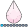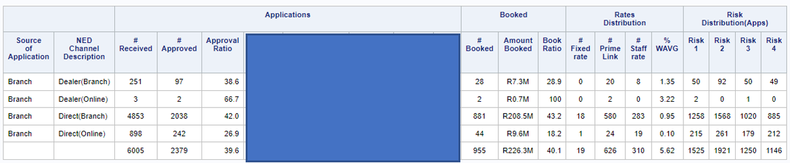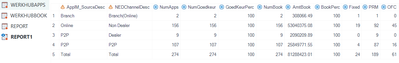Hi,

i am really struggling with a code, I have all the fields that needs to be used in the code.

the code should have the specific layout as per the screen print attached. i have attached the code Aswell. please can someone help me to solve this report.

5 REPLIES 5HB
Barite | Level 11

Generally, with the threat of viruses and malware, nobody is going to open a .docx attachment.  I'm not.

Put a functional sample of your data in a working data step, post that, post the code you have written, and fully describe what you want (with example output!) and you will be MUCH more likely to get help.

If you have a picture or screen capture of your table you can attach an image using the camera icon that appears above the message box.

But likely some description will be needed, at least variable types and names. DATA step code to recreate some data is preferred because then we don't have to ask about things like variable type and we have something to test.

@MJM11111 You are sharing a lot of code and it's at least to me not clear with which step you are struggling with.

Questions

1. Is the code you've shared your actual code or just some "sample"
2. Does the code you've shared execute without errors and warnings in your environment?
3. Do you know how to shape the data so it's suitable input for Proc Report?
4. Do you manage to shape the data in this way?
5. Is the picture of a report you share the desired outcome or what your Proc Report currently creates?

You need to split your big problem into smaller chunks and then solve them one by one. Some of above questions will hopefully give you a start to do so.

Most of us will also need some representative sample Have data to help you with your code which means you will have to create such sample data and share it by posting a data step that creates it.

Below and attached the content of your Word doc.1st I need to get all the total number off accounts received , then what was approved.

All need to linked back to the Source off application ( 33,34,35) , then as I understand I need to merge two summary table where I counted the number off account that come in, the total number approved , the number booked, those needs to also be linked back to the fixed rate, prime rate and staff rate, together with the risk1,risk2, risk3,risk4.

## Calculations not pulling through correctly.

Hi,

I have this code attached below.

the outcome on the report needs to be as follow:

NumApp =2166

NumGoedKeur =300

Numbook =274

I managed to get the Approved Applications that off 274, but the problem comes in where the NumApp and the NumGoedkeur is the same as 274, and it actually needs to be NumApp= 2166, and the NumGoedKeur is 300. so somewhere in my code i am not calculating the correct table in order to get the NumApps and the NumGoedkeur, and due to the  NumApps, NumGoedkeur and Numbook is pulling through the same amount of 274, the calculations off all the other fields is showing 100%please can someone please help me just to sort out this small calculation mistake then i can create the rest of the report.

Proc sql;
create table data1 as
select A.KeyDateEntr,a.Applm_StatusCode, a.ApplM_Status,a.ApplM_MarketCode,a.ApplM_MarketName,
a.ApplM_Prindebt,A.Applm_NedChannel,a.ApplM_Source,a.Applm_SourceDesc,
B.KeyDateBook,b.AccoM_RepStatus,b.AccoM_MotorDiv, b.AccoM_RateOffset,
b.AccoM_RateType,b.MFCRef,b.AccoM_Rate, b.AccoM_Prindebt,b.monthbook
from WerkWM.MartAppMini as a
left join werkwm.martaccount as b
on a.KeyIBIS = b.KeyIBIS
where b.monthbook = 202308
and  ApplM_Source in ('32', '33', '34');
quit;

Data data2;
set data1;
if KeyDateEntr ne . then AppCount = 1;
if ApplM_StatusCode = 'CAPD' then Goedkeur = 1;
if KeyDatebook ne . then NumBook = 1;
if AccoM_RateType = "PRM" then PRM = 1;
else if AccoM_RateType = "FXD" then Fixed  = 1;
else if AccoM_RateType = "OFC" then OFC = 1;

Attrib NEDChannelDesc format = \$25. label = 'NED Channel Description';
NEDChannelDesc = put(ApplM_NedChannel,NEDChannelDesc.);
run;
proc sort data = data2;
by KeyAInfra;
run;
data data3(drop = KeyAInfra);
merge data2(in = hoof)
WerkWM.DIMAPPLINFRA(keep = KeyApplInfraM ApplM_ExternalRef rename = (KeyApplInfraM = KeyAInfra));
by KeyAInfra;
if hoof;

if ApplM_Source = '32' then do;
if missing(ApplM_ExternalRef) then NEDChannelDesc = catt(NEDChannelDesc,'(Online)');
else NEDChannelDesc = catt(NEDChannelDesc,'(Branch)');
end;
run;
Proc Sql;
Create Table WerkHubApps as
select Applm_SourceDesc,NEDChannelDesc,
sum(AppCount) as NumApps ,
sum(Goedkeur) as NumGoedkeur,
sum(case when ApplM_SCategory = "Risk1" then 1 else 0 end) as Risk1 format = 8.,
sum(case when ApplM_SCategory = "Risk2" then 1 else 0 end) as Risk2 format = 8.,
sum(case when ApplM_SCategory = "Risk3" then 1 else 0 end) as Risk3 format = 8.,
sum(case when ApplM_SCategory = "Risk4" then 1 else 0 end) as Risk4 format = 8.
from data3
group by Applm_SourceDesc, NEDChannelDesc

UNION ALL

select "Total" as Applm_SourceDesc,"Total" as NEDChannelDesc,
sum(AppCount) as NumApps ,
sum(Goedkeur) as NumGoedkeur,
sum(case when ApplM_SCategory = "Risk1" then 1 else 0 end) as Risk1 format = 8.,
sum(case when ApplM_SCategory = "Risk2" then 1 else 0 end) as Risk2 format = 8.,
sum(case when ApplM_SCategory = "Risk3" then 1 else 0 end) as Risk3 format = 8.,
sum(case when ApplM_SCategory = "Risk4" then 1 else 0 end) as Risk4 format = 8.
from data3;

create table WerkHubBook as
select Applm_SourceDesc,NEDChannelDesc,
sum(NumBook) as NumBook,
sum(AccoM_Prindebt) as AmtBook format = KRandm.,
sum(Fixed) as Fixed label = "# Fixed Rate",
sum(PRM) as PRM label = "# Prime Link",
sum(OFC) as OFC label = "# Staff Rate",
sum(AccoM_RateOffset*AccoM_Prindebt) as AmtRatWei,
sum(AccoM_Prindebt) as AmtBook1 format = 15.2,
calculated AmtRatWei / calculated AmtBook as WAVG format = 6.2
from data3
where NumBook = 1
group by Applm_SourceDesc, NEDChannelDesc

UNION ALL

select "Total" as Applm_SourceDesc,"Total" as NEDChannelDesc,
sum(NumBook) as NumBook,
sum(AccoM_Prindebt) as AmtBook format = KRandm.,
sum(Fixed) as Fixed label = "# Fixed Rate",
sum(PRM) as PRM label = "# Prime Link",
sum(OFC) as OFC label = "# Staff Rate",
sum(AccoM_RateOffset*AccoM_Prindebt) as AmtRatWei,
sum(AccoM_Prindebt) as AmtBook1 format = 15.2,
calculated AmtRatWei / calculated AmtBook as WAVG format = 6.2
from data3
where NumBook = 1;

quit;

/*Building the report*/

Data Report;
merge werkhubApps WerkHubBook;
Attrib GoedKeurPerc format = 4.1    Label = "Approval Ratio"
BookPerc     format = 4.1    Label = "Book Ratio"
AmtBook      format = KRandm.;
by Applm_SourceDesc NEDChannelDesc;
if NumApps > 0 then GoedKeurPerc = round(NumGoedkeur / NumApps * 100,0.1);
else GoedKeurPerc = 0;
if NumGoedkeur > 0 then BookPerc = round(NumBook / NumGoedkeur * 100,0.1);
else BookPerc = 0;
if missing(Fixed)  then Fixed = 0;
if missing(PRM) then PRM = 0;
if missing(OFC) then OFC = 0;
if missing(NEDChannelDesc) then delete;
run;

thank you

## Re: Calculations not pulling through correctly.

I moved this back in here, where it belongs.

Please post a usable (DATA step with DATALINES) example of data1 and WerkWM.DIMAPPLINFRA, so we have something to play around with. Also post the expected outcome for this example data.

Discussion stats
• 5 replies
• 369 views
• 0 likes
• 5 in conversation